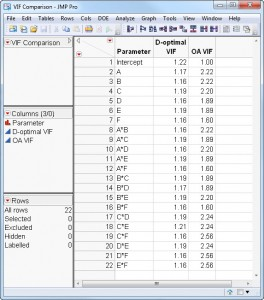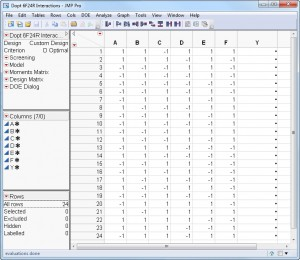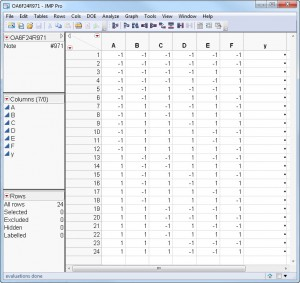Our World Statistics Day conversations have been a great reminder of how much statistics can inform our lives. Do you have an example of how statistics has made a difference in your life? Share your story with the Community!
Choose Language Hide Translation Bar
What price orthogonality?

On Feb. 8, my colleague, Professor Douglas Montgomery of Arizona State University, and I presented a webinar for the American Statistical Association. Our first demonstration dealt with designing an experiment for six factors each having two levels in 24 runs. One natural way to construct such a design would be to choose six columns from the orthogonal array discovered by Plackett and Burman, which has 24 rows and 23 columns (see below).

+++++++++++++++++++++++

−++++−+−++−−++−−+−+−−−−

−−++++−+−++−−++−−+−+−−−

−−−++++−+−++−−++−−+−+−−

−−−−++++−+−++−−++−−+−+−

−−−−−++++−+−++−−++−−+−+

+−−−−−++++−+−++−−++−−+−

−+−−−−−++++−+−++−−++−−+

+−+−−−−−++++−+−++−−++−−

−+−+−−−−−++++−+−++−−++−

−−+−+−−−−−++++−+−++−−++

+−−+−+−−−−−++++−+−++−−+

++−−+−+−−−−−++++−+−++−−

−++−−+−+−−−−−++++−+−++−

−−++−−+−+−−−−−++++−+−++

+−−++−−+−+−−−−−++++−+−+

++−−++−−+−+−−−−−++++−+−

−++−−++−−+−+−−−−−++++−+

+−++−−++−−+−+−−−−−++++−

−+−++−−++−−+−+−−−−−++++

+−+−++−−++−−+−+−−−−−+++

++−+−++−−++−−+−+−−−−−++

+++−+−++−−++−−+−+−−−−−+

++++−+−++−−++−−+−+−−−−−

Hold on a sec. What is an orthogonal array?

An orthogonal array is a matrix of symbols, in our case “+” and “–“. In each pair of columns, there are only four possible pairs of symbols: “+ +”, “+ –“, ”– +” and “– –“. To be an orthogonal array, each pair of symbols has to occur equally often in every pair of columns. In the above array, each of the four pairs of symbols appears six times in every one of the 253 pairs of columns.

Orthogonal arrays have great historical significance in the field of experiment design. Until the advent of computers, virtually every experiment design used an orthogonal array. There are two main reasons why early practitioners focused on orthogonal arrays. First, it is easy to calculate the effect of changing any factor – you just average all the responses for trials run at the + level of the factor and subtract the average of all the response for trials run at the – level of the factor. Second, the estimated effect of any factor is statistically independent of the estimated effect of any other factor.

If orthogonal arrays are so great, why use anything else?

Orthogonal arrays are very useful, but they only exist for certain numbers of trials. For example, orthogonal arrays having two symbols in each column only exist when the number of trials is a multiple of four. If all the factors have two levels, an orthogonal array with 15 rows does not exist.

More important is the fact that although the main effects of each factor in an orthogonal array are independent, the two-factor interactions may not be. In the example that Doug and I presented, we wanted to be able to estimate both the six main effects as well as the 15 two-factor interactions. If you include the overall average, there are 22 unknown quantities that we want to estimate. Since there are 24 runs, it seems possible to fit this 22-term model using ordinary least squares.

Can you choose six columns from the Plackett-Burman design to fit this model?

It depends on which six columns you use. There are 100,947 ways you can pick six columns out of the 23. Of those, more than half are incapable of fitting the two-factor interactions model. Of course, there are 49,588 ways of choosing the six columns that do allow for fitting all the two-factor interactions. Depending on which group of six columns you choose, the average variance of the coefficient estimates can vary by a factor of >28. That is, for the six-column design, the least desirable choice estimates the coefficients 28 times worse than the most desirable choice. So, to construct your design by picking six columns from the above array, you have to be very careful.

Are there other orthogonal design choices that are better?

I asked my colleague, Dr. Eric Schoen of the University of Antwerp, for help here. Eric is a world-renowned researcher in orthogonal design. He has constructed a catalog of all the statistically different orthogonal arrays having six columns and 24 rows. It turns out that there are only 1,350 of them. So, most of the six column choices from the 23 columns of the Plackett-Burman design were non-unique. Also, they are not exhaustive. It turns out that 20 of Eric’s orthogonal arrays were better than any of the six column choices from the Plackett-Burman design. The best of these was about 8% better than the best orthogonal array I found previously. Only 447 of the 1,350 unique orthogonal arrays could fit the model.

Can you do better at estimating all the effects of interest?

The answer is yes but only if you do not use an orthogonal array! I constructed a D-optimal design using the Custom Designer in JMP. Table 1 shows the comparison of the variance inflation factors (VIF) for the D-optimal design compared to the best orthogonal array.Note that the VIF for every main effect and two-factor interaction is lower (better) for the D-optimal design than for the best of the orthogonal arrays.

The bottom line – don’t limit yourself by only considering orthogonal arrays.

Many investigators only consider orthogonal arrays when planning their experiments. This restriction comes at a price as the example from the webinar shows.

Finding the best orthogonal array actually required more experiment design expertise and more computing than finding the D-optimal design, and the resulting design was statistically inferior.Article Labels

There are no labels assigned to this post.

Article Tags